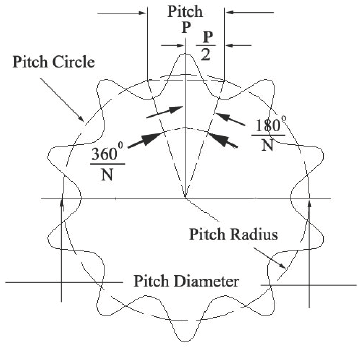Related Resources: calculators

### Sprocket Pitch Diameter Formulas and Calculator

Gear Design and Selection Application

Sprocket Pitch Diameter Formulas and Calculator

The sprocket pitch diameter is an imaginary circle through which the chain pin centers move around the sprocket. The pitch diameter is the fundamental design geometry that determines the size shape and form of the sprocket teeth dimensions.Sprocket Pitch Circle Diameter Equation

PD = P / sin [ 180° / N ]

Where:

PD = Pitch Diameter
P = Chain Pitch in inches
N = Number of teeth on the sprocket

Related:

Reference ANSI/ASME B29.1M